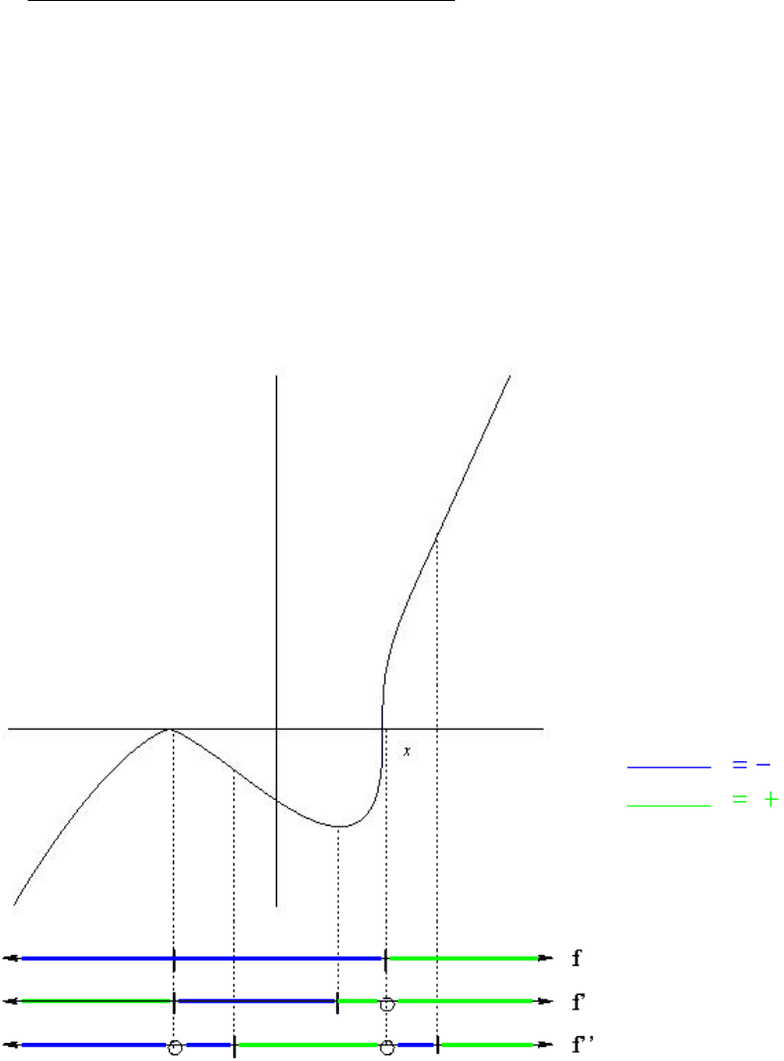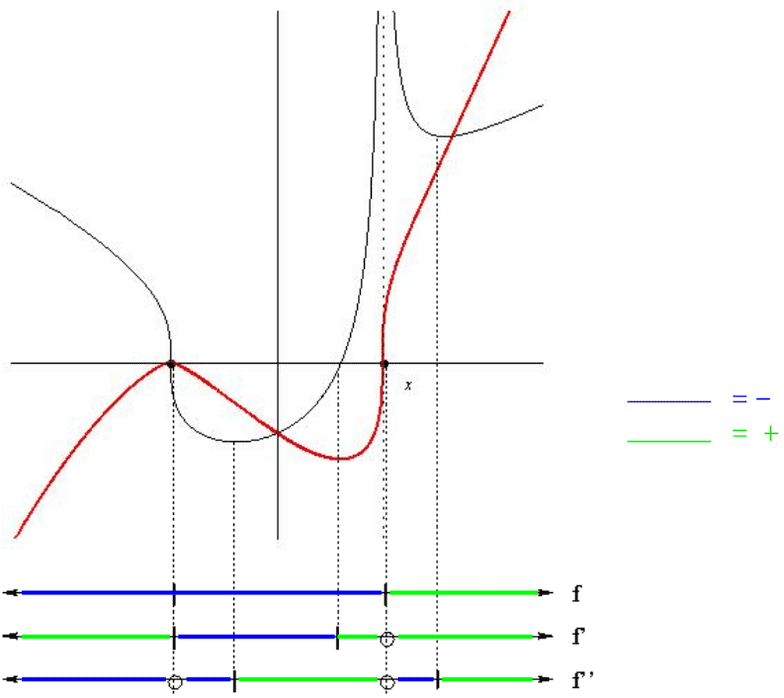# MTH 151 Midterm: MTH151 Term Test 3 2010 Spring Solutions

53 views5 pagesName:
Exam 2 - Math 151
April 12, 2010
R. Ketchersid
Instructions. You may use a calculator, but I want exact answers on each question. You must
show work for credit, you may perform scratch work on a separate sheet, but everything you want
graded should be on this exam. For this exam you need not (and probably should not) simplify the
expressions you get.
1. [5pts] Below is the graph of f(x), I want you to ﬁll in the sign diagrams for f(x),f0(x), and
f00 (x). (Make the information on the sign diagrams match up as close as possible with the graph.)
Graph of f(x)
Unlock document

This preview shows pages 1-2 of the document.
Unlock all 5 pages and 3 million more documents.-2-
2. [5pts] Below is the graph of f0(x), I want you to ﬁll in the sign diagrams for f0(x)and f00 (x).
Then use this information to construct a graph of f(x)and ﬁll out the sign diagram for f(x). The
two displayed points are the roots of f(x)and f(x)is continuous everywhere.
Graph of f0(x)
Unlock document

This preview shows pages 1-2 of the document.
Unlock all 5 pages and 3 million more documents.

## Document Summary

You may use a calculator, but i want exact answers on each question. You must show work for credit, you may perform scratch work on a separate sheet, but everything you want graded should be on this exam. For this exam you need not (and probably should not) simplify the expressions you get. [5pts] below is the graph of f(x), i want you to ll in the sign diagrams for f(x), f(cid:48)(x), and. 1. f(cid:48)(cid:48)(x). (make the information on the sign diagrams match up as close as possible with the graph. ) [5pts] below is the graph of f(cid:48)(x), i want you to ll in the sign diagrams for f(cid:48)(x) and f(cid:48)(cid:48)(x). Then use this information to construct a graph of f(x) and ll out the sign diagram for f(x). The two displayed points are the roots of f(x) and f(x) is continuous everywhere.